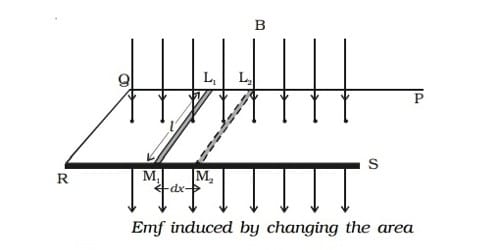# Explain Methods of Producing Induced emf

Electromotive force (EMF) is the characteristic of any energy source capable of driving electric charge around a circuit. It is abbreviated E in the international metric system but also, popularly, as emf. According to Faraday’s law of electromagnetic induction, an emf is induced in a circuit when magnetic flux linked with it changes. This emf is called induced emf.

Methods of producing induced emf

We know that the induced emf is given by the expression

e = – dφ/dt = – d/dt

= − (NBA cos θ)

Hence, the induced emf can be produced by changing

(i) the magnetic induction (B)

(ii) the area enclosed by the coil (A) and

(iii) the orientation of the coil (θ) with respect to the magnetic field.

For Example, Emf induced by changing the magnetic induction. The magnetic induction can be changed by moving a magnet either towards or away from a coil and thus an induced emf is produced in the coil. Suppose, initially the coil is in the vertical position so that the angle between normal to the plane of the coil and magnetic field is zero. The magnetic induction can also be changed in one coil by changing the current in the neighboring coil thus producing an induced emf.

Some examples of energy source which provide emf are electrochemical cells, thermoelectric devices, solar cells, and electrical generators.